# Newtons Laws Newtons First Law of Motion Every

• Slides: 43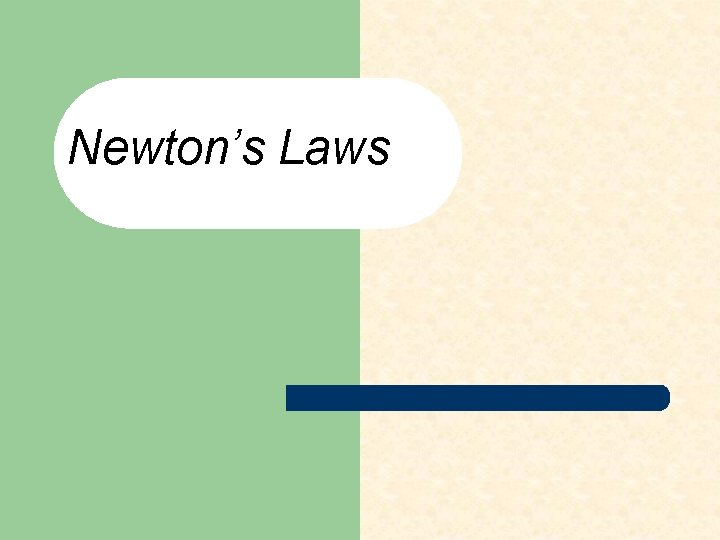Newton’s Laws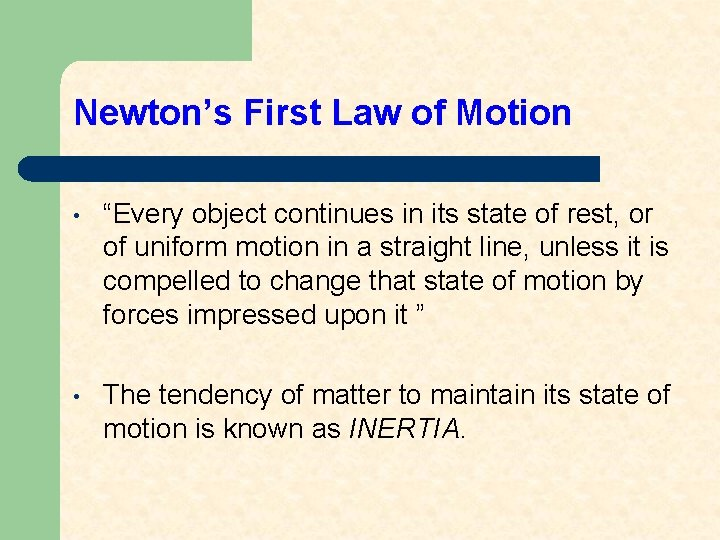Newton’s First Law of Motion • “Every object continues in its state of rest, or of uniform motion in a straight line, unless it is compelled to change that state of motion by forces impressed upon it ” • The tendency of matter to maintain its state of motion is known as INERTIA.Newton’s First Law of Motion • Inertia prevents an object at rest from moving spontaneously or an object in uniform linear motion from changing its speed or direction spontaneously. • Force is simply a push or a pull.Newton’s First Law of Motion • Changes in motion do not happen spontaneously. • Example: 1) Tablecloth under dishesNewton’s First Law of Motion Inertia is the tendency of an object to resist changes in its velocity: whether in motion or motionless. These pumpkins will not move unless acted on by an unbalanced force.Forces • • • Usually think of a force as a push or pull Vector quantity May be a contact force or a field force • • Contact forces result from physical contact between two objects Field forces act between disconnected objects • Also called “action at a distance”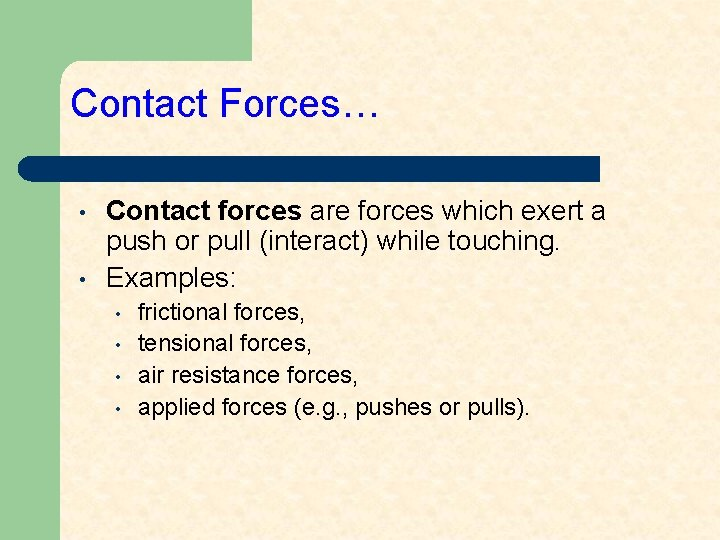Contact Forces… • • Contact forces are forces which exert a push or pull (interact) while touching. Examples: • • frictional forces, tensional forces, air resistance forces, applied forces (e. g. , pushes or pulls).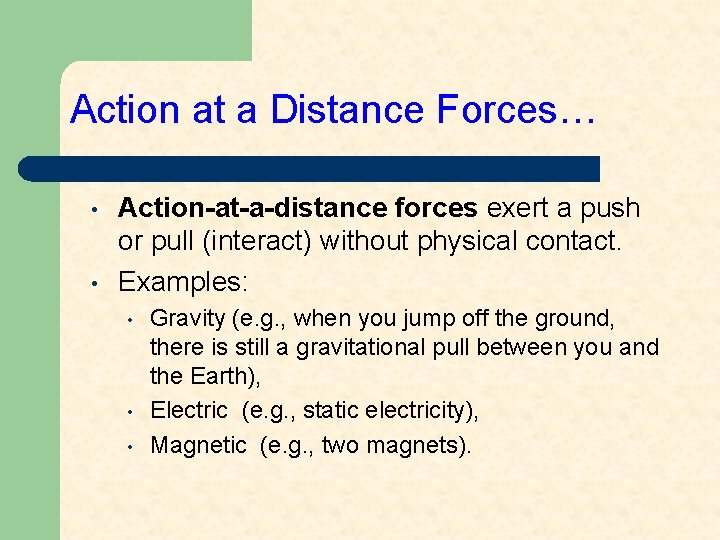Action at a Distance Forces… • • Action-at-a-distance forces exert a push or pull (interact) without physical contact. Examples: • • • Gravity (e. g. , when you jump off the ground, there is still a gravitational pull between you and the Earth), Electric (e. g. , static electricity), Magnetic (e. g. , two magnets).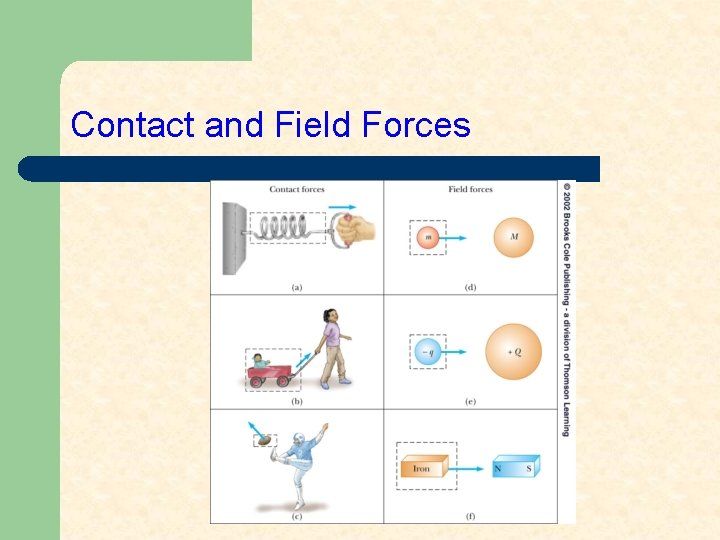Contact and Field Forces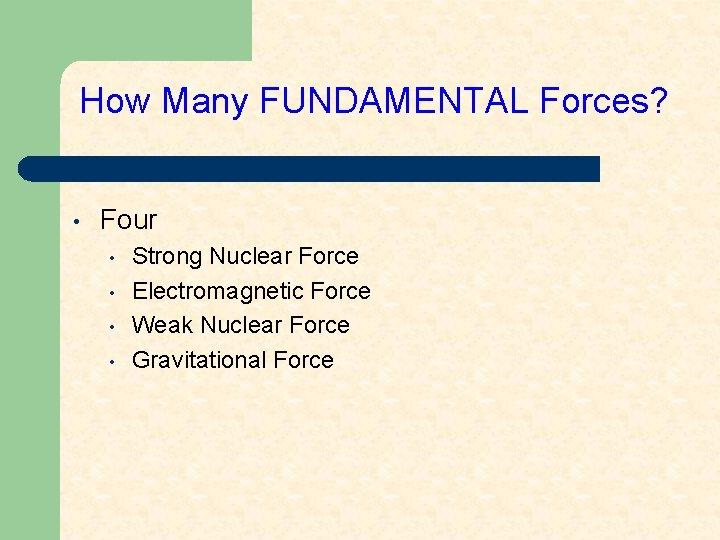How Many FUNDAMENTAL Forces? • Four • • Strong Nuclear Force Electromagnetic Force Weak Nuclear Force Gravitational Force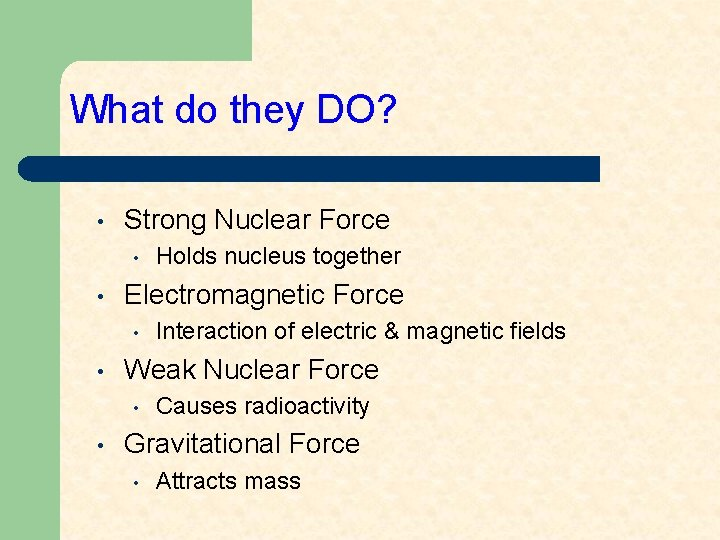What do they DO? • Strong Nuclear Force • • Electromagnetic Force • • Interaction of electric & magnetic fields Weak Nuclear Force • • Holds nucleus together Causes radioactivity Gravitational Force • Attracts mass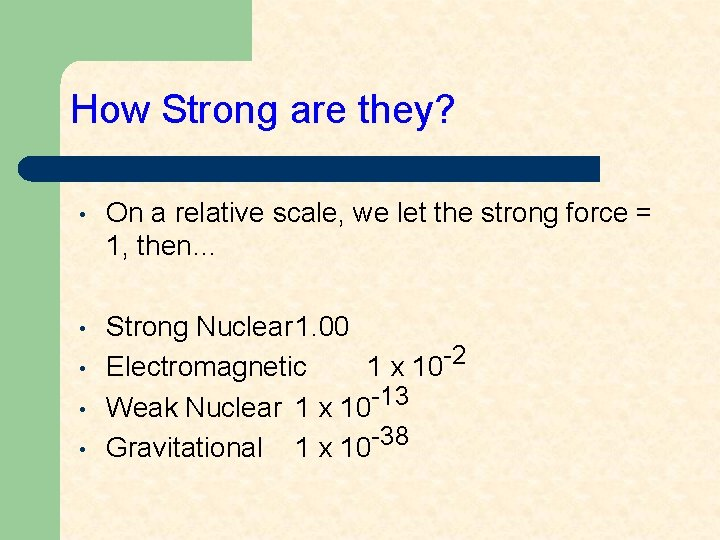How Strong are they? • On a relative scale, we let the strong force = 1, then… • Strong Nuclear 1. 00 Electromagnetic 1 x 10 -2 Weak Nuclear 1 x 10 -13 Gravitational 1 x 10 -38 • • •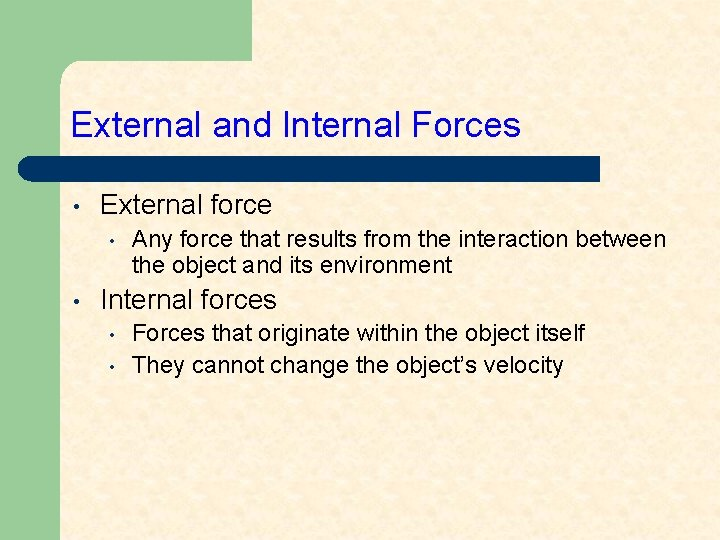External and Internal Forces • External force • • Any force that results from the interaction between the object and its environment Internal forces • • Forces that originate within the object itself They cannot change the object’s velocityExample When the ball at the end of the string swings to its lowest point, the string is cut by a sharp razor. Which path will the ball then follow?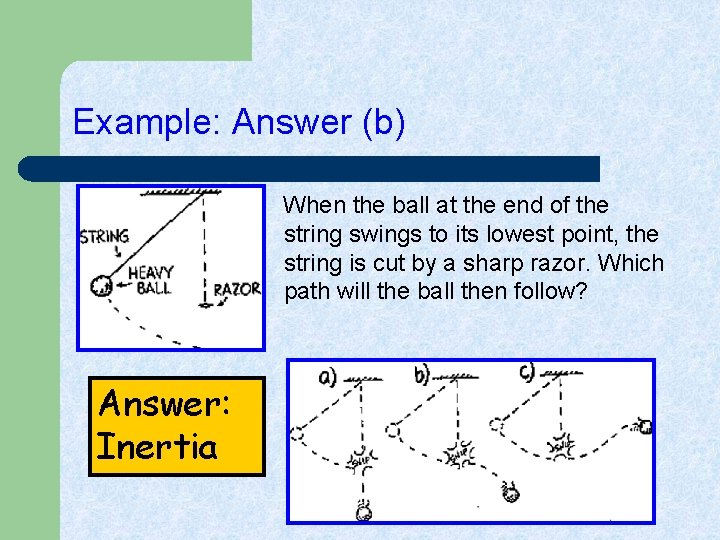Example: Answer (b) When the ball at the end of the string swings to its lowest point, the string is cut by a sharp razor. Which path will the ball then follow? Answer: Inertia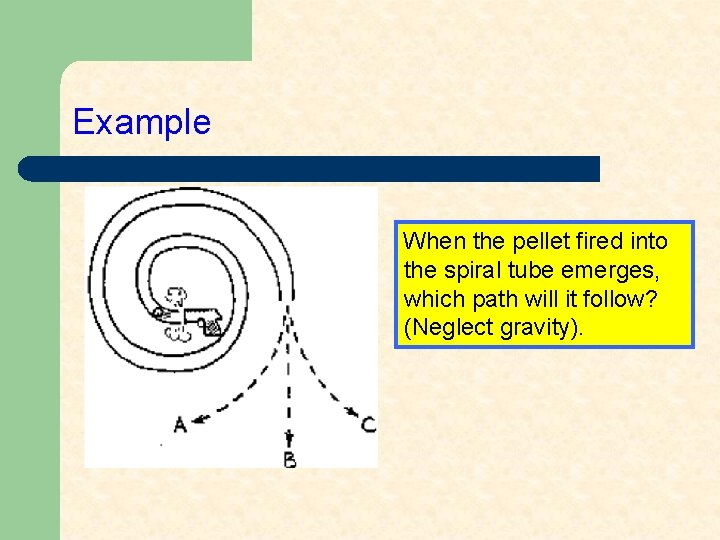Example When the pellet fired into the spiral tube emerges, which path will it follow? (Neglect gravity).Example: Answer (b) When the pellet fired into the spiral tube emerges, which path will it follow? (Neglect gravity). Answer: InertiaNewton’s Second Law of Motion • A commonsense law. • The force on an object is equal to its mass multiplied by its acceleration. • Mass is the amount of matter an object has. • Acceleration is the time rate of change of velocity. (Velocity is speed in a given direction. )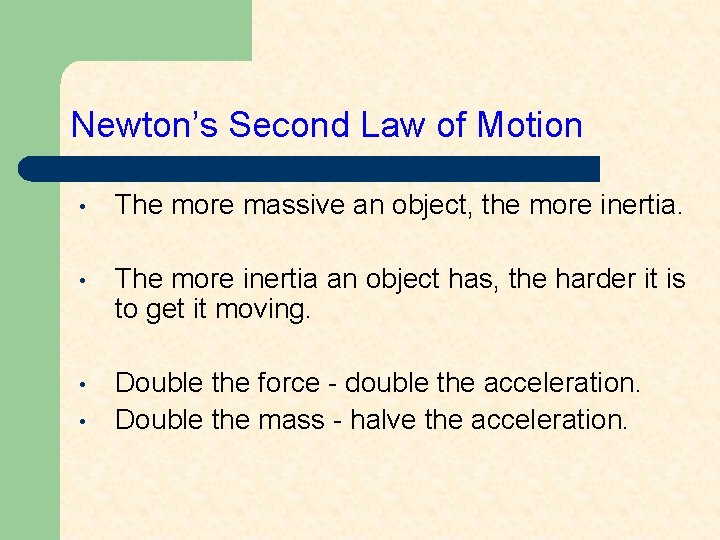Newton’s Second Law of Motion • The more massive an object, the more inertia. • The more inertia an object has, the harder it is to get it moving. • Double the force - double the acceleration. Double the mass - halve the acceleration. •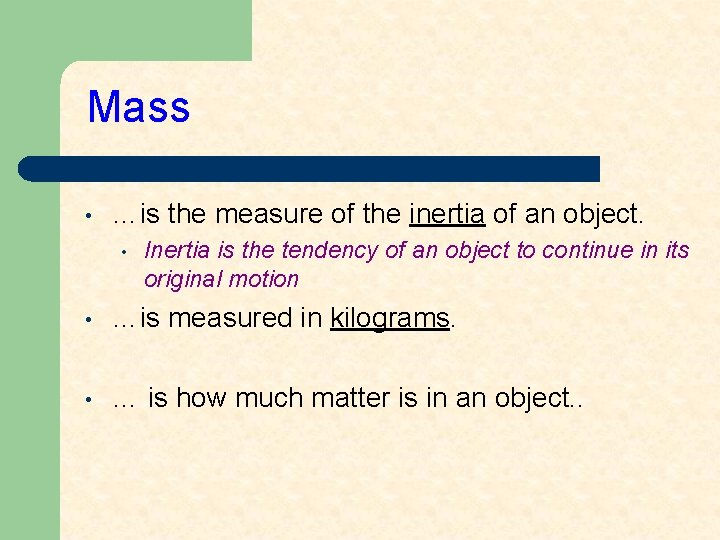Mass • …is the measure of the inertia of an object. • Inertia is the tendency of an object to continue in its original motion • …is measured in kilograms. • … is how much matter is in an object. .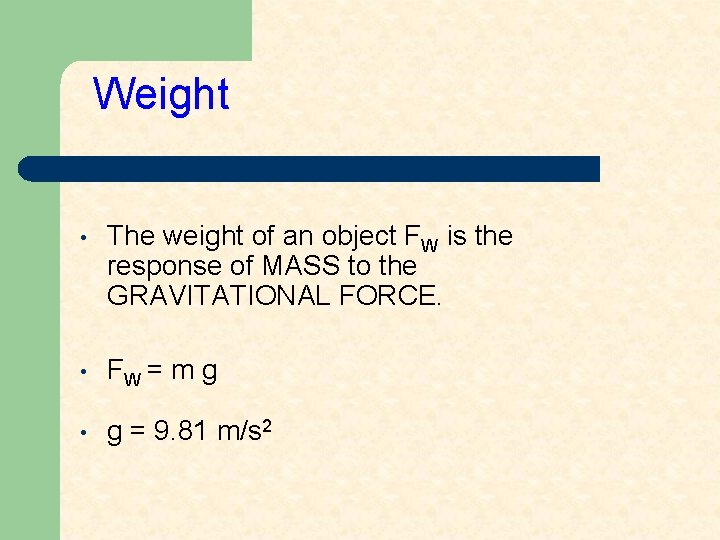Weight • The weight of an object FW is the response of MASS to the GRAVITATIONAL FORCE. • FW = m g • g = 9. 81 m/s 2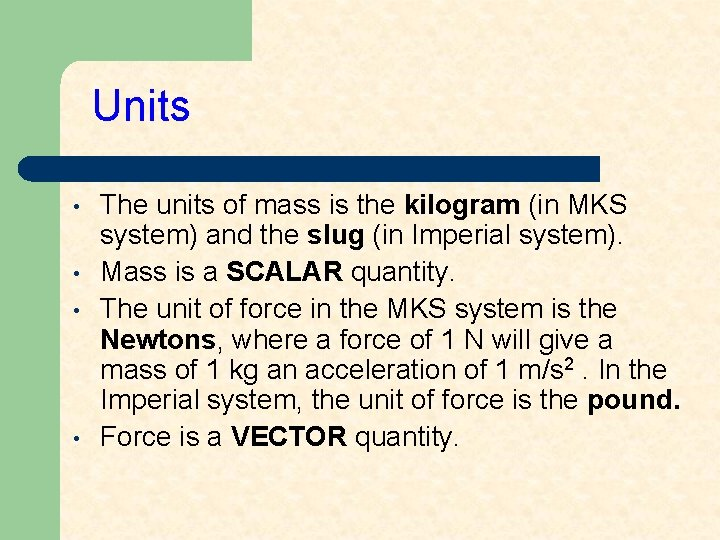Units • • The units of mass is the kilogram (in MKS system) and the slug (in Imperial system). Mass is a SCALAR quantity. The unit of force in the MKS system is the Newtons, where a force of 1 N will give a mass of 1 kg an acceleration of 1 m/s 2. In the Imperial system, the unit of force is the pound. Force is a VECTOR quantity.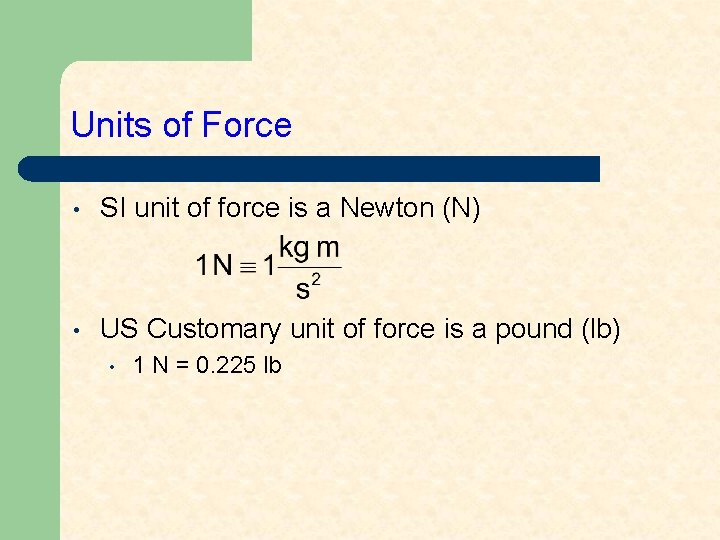Units of Force • SI unit of force is a Newton (N) • US Customary unit of force is a pound (lb) • 1 N = 0. 225 lb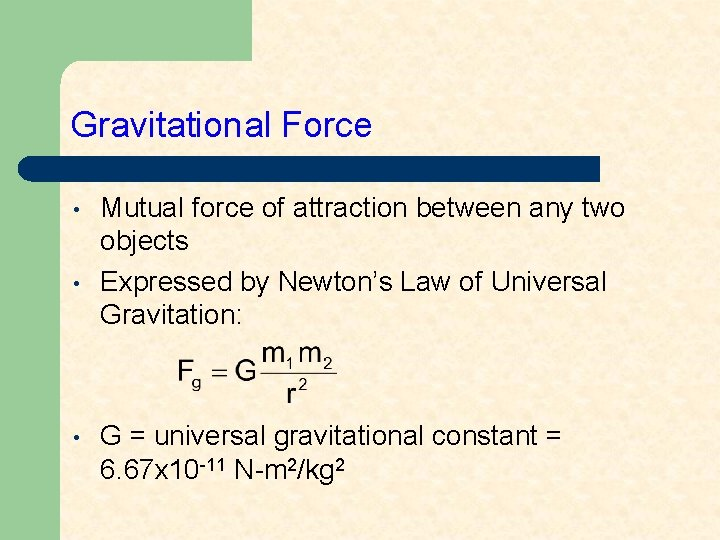Gravitational Force • • • Mutual force of attraction between any two objects Expressed by Newton’s Law of Universal Gravitation: G = universal gravitational constant = 6. 67 x 10 -11 N-m 2/kg 2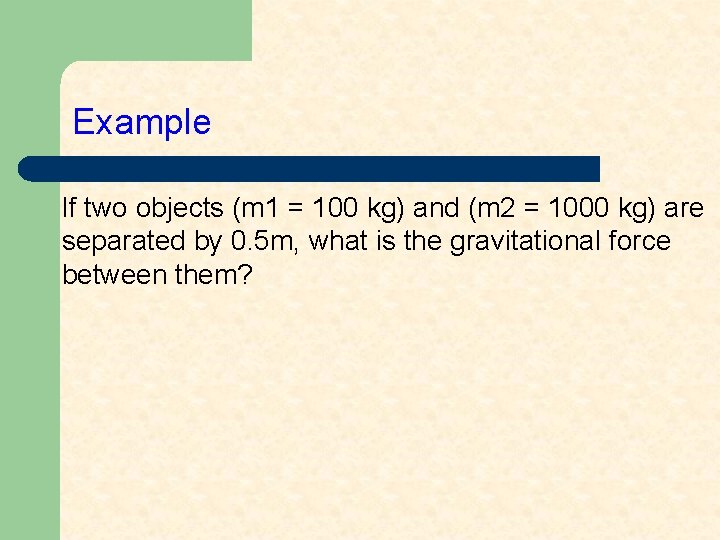Example If two objects (m 1 = 100 kg) and (m 2 = 1000 kg) are separated by 0. 5 m, what is the gravitational force between them?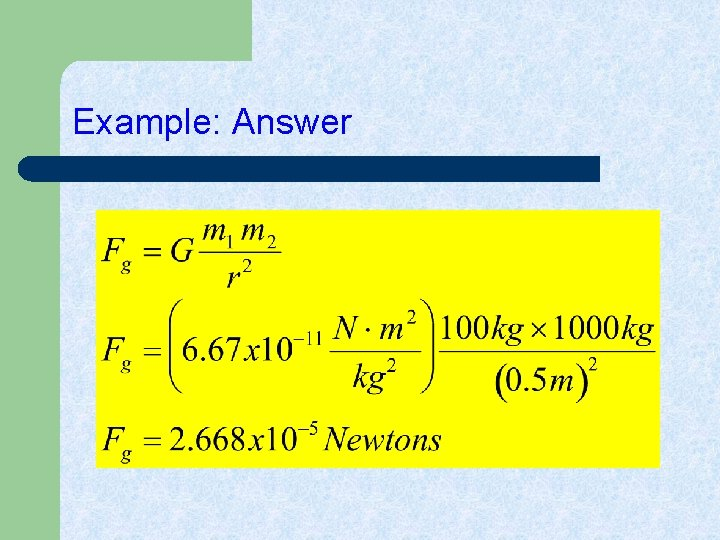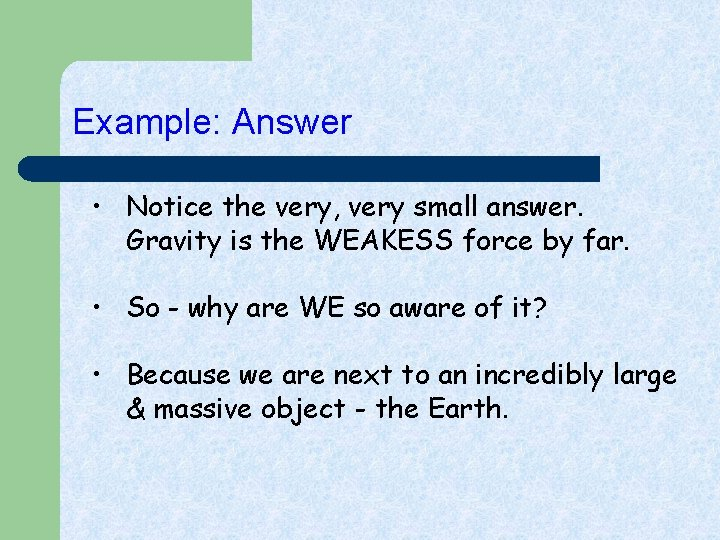Example: Answer • Notice the very, very small answer. Gravity is the WEAKESS force by far. • So - why are WE so aware of it? • Because we are next to an incredibly large & massive object - the Earth.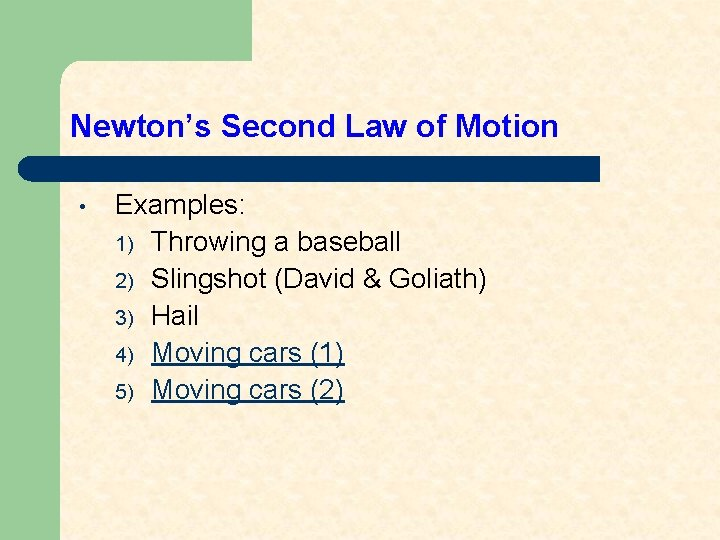Newton’s Second Law of Motion • Examples: 1) Throwing a baseball 2) Slingshot (David & Goliath) 3) Hail 4) Moving cars (1) 5) Moving cars (2)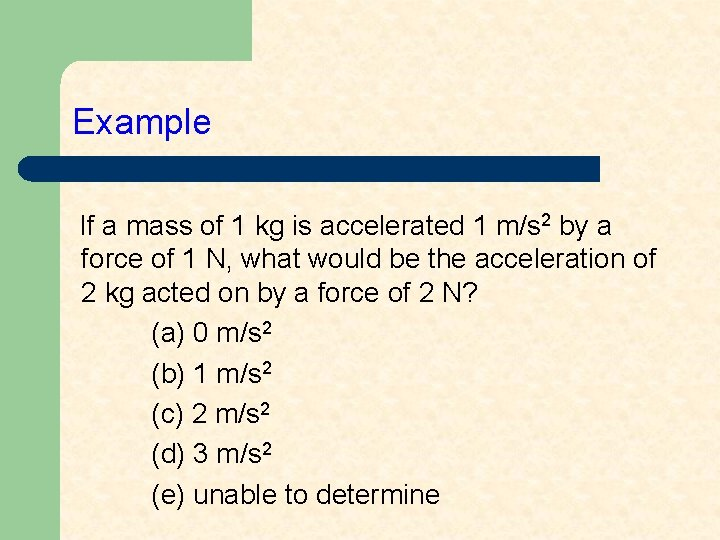Example If a mass of 1 kg is accelerated 1 m/s 2 by a force of 1 N, what would be the acceleration of 2 kg acted on by a force of 2 N? (a) 0 m/s 2 (b) 1 m/s 2 (c) 2 m/s 2 (d) 3 m/s 2 (e) unable to determine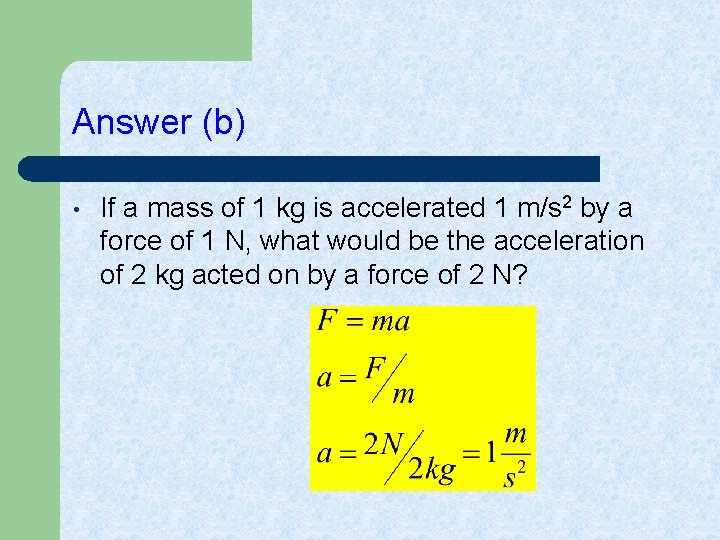Answer (b) • If a mass of 1 kg is accelerated 1 m/s 2 by a force of 1 N, what would be the acceleration of 2 kg acted on by a force of 2 N?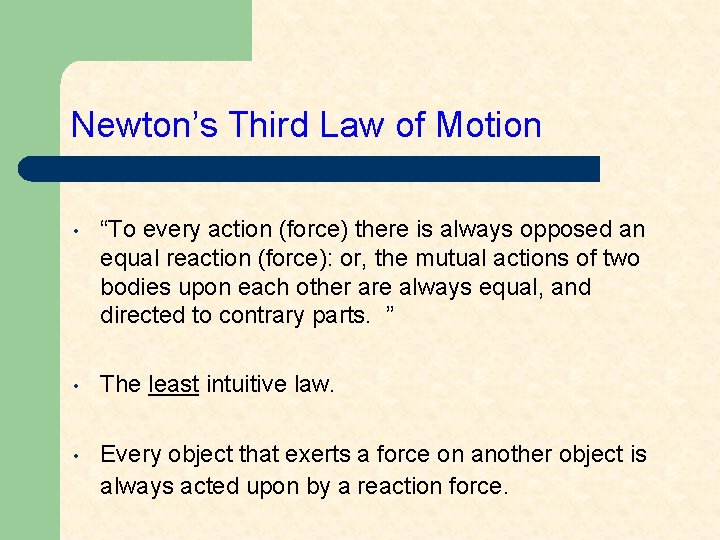Newton’s Third Law of Motion • “To every action (force) there is always opposed an equal reaction (force): or, the mutual actions of two bodies upon each other are always equal, and directed to contrary parts. ” • The least intuitive law. • Every object that exerts a force on another object is always acted upon by a reaction force.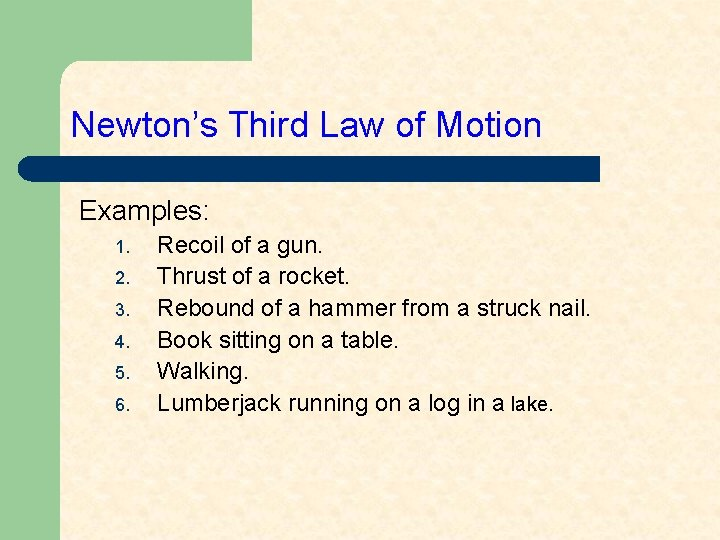Newton’s Third Law of Motion Examples: 1. 2. 3. 4. 5. 6. Recoil of a gun. Thrust of a rocket. Rebound of a hammer from a struck nail. Book sitting on a table. Walking. Lumberjack running on a log in a lake.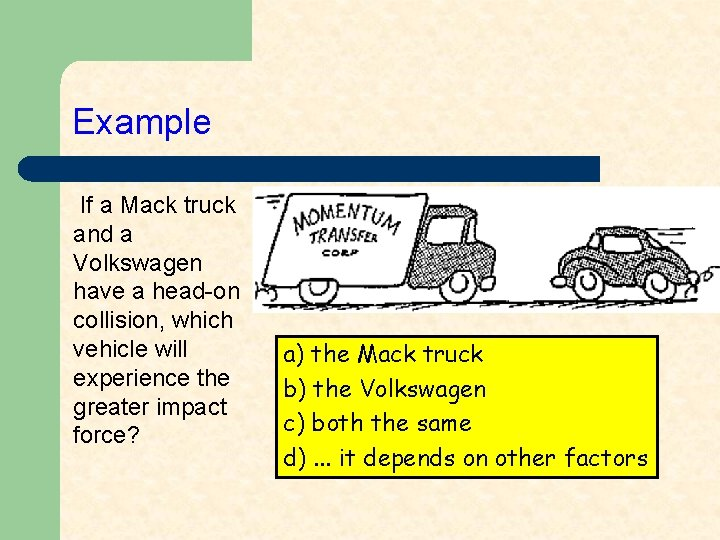Example If a Mack truck and a Volkswagen have a head-on collision, which vehicle will experience the greater impact force? a) the Mack truck b) the Volkswagen c) both the same d). . . it depends on other factorsExample: Answer (c) If a Mack truck and a Volkswagen have a head-on collision, which vehicle will experience the greater impact force? Answer: Newton’s Third Law ~ action and reactionExample Consider the apple at rest on the table. If we call the gravitational force exerted on the apple action, what is the reaction force according to Newton's 3 rd Law?Example: Answer Consider the apple at rest on the table. If we call the gravitational force (by the earth) exerted on the apple action, what is the reaction force according to Newton's 3 rd Law? Answer: The gravitational force exerted by the apple on the earthExample If a mass of 10 kilograms is accelerated at 5 meters per second, what is the force acting on it?Example What acceleration will result when a 12 -N net force applied to a 3 -kg object? (a) 0 m/s 2 (b) 4 m/s 2 (c) 36 m/s 2 (d) Unable to determine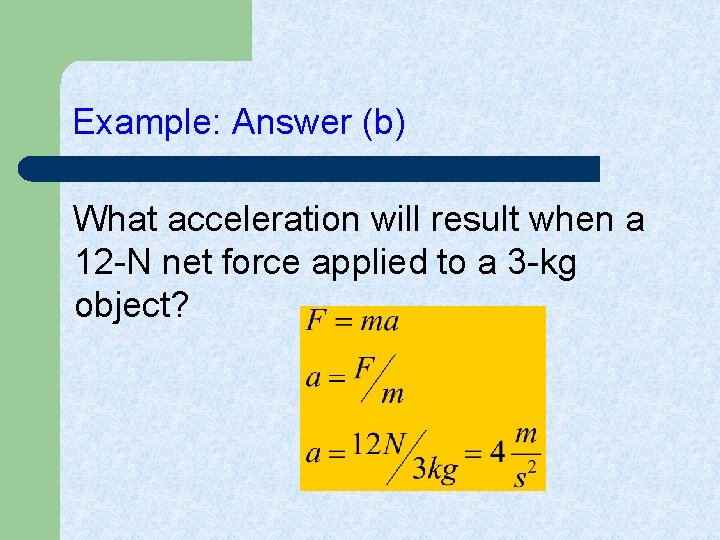Example: Answer (b) What acceleration will result when a 12 -N net force applied to a 3 -kg object?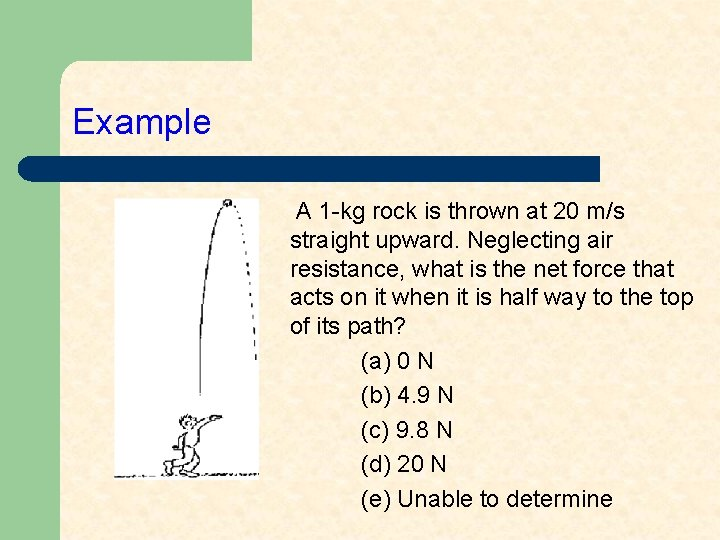Example A 1 -kg rock is thrown at 20 m/s straight upward. Neglecting air resistance, what is the net force that acts on it when it is half way to the top of its path? (a) 0 N (b) 4. 9 N (c) 9. 8 N (d) 20 N (e) Unable to determineAnswer (c) Answer: The only force acting on the rock is gravity. F=ma F = (1) (9. 8)=9. 8 N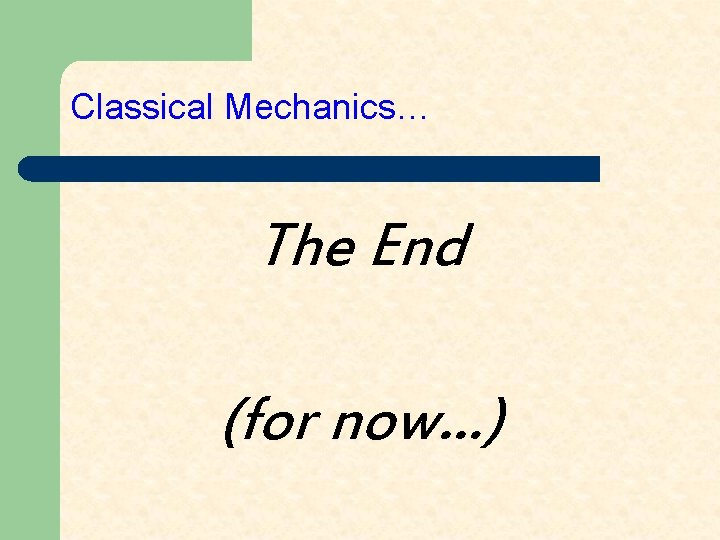Classical Mechanics… The End (for now…)